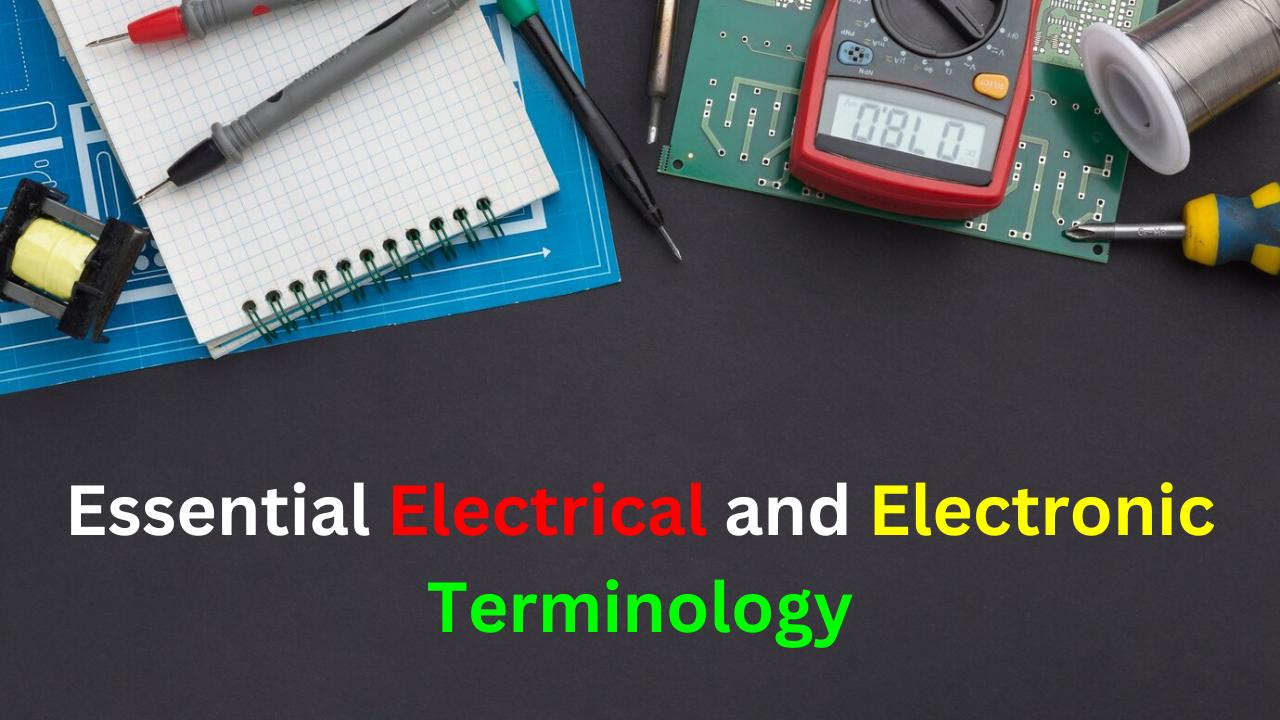## Essential Electrical and Electronic TerminologyAh Rating: A capacity rating for batteries calculated by multiplying current (A) by the length of time (h) a battery can deliver that current to a load.

Ammeter: An instrument used to measure electrical current.

Ampere (A): The unit of electrical current.

Atom: The smallest particle of an element with its unique characteristics.

AWG (American Wire Gauge): A standardization based on wire diameter.

Charge: An electrical property resulting from an excess or deficiency of electrons; can be positive or negative.

Circuit Breaker: A resettable protective device for interrupting excessive current.

Circuit: An interconnection of electrical components designed for a specific purpose.

Closed Circuit: A circuit with a complete current path.

Conductance: The ability of a circuit to allow current; measured in Siemens (S).

Conductor: A material where electric current is easily established, e.g., copper.

Coulomb (C): The unit of electrical charge; the charge possessed by 6.25 x 10^18 electrons.

Coulomb’s Law: States a force between charged bodies is directly proportional to the product of charges and inversely proportional to the square of the distance between them.

Current Source: A device producing a constant current for a varying load.

Current: The rate of flow of charge (free electrons).

DMM (Digital Multimeter): An electronic instrument measuring voltage, current, and resistance.

Efficiency: The ratio of the output power to the input power of a circuit, usually expressed as a percentage.

Electron: A basic particle of negative electrical charge in matter.

Energy: The ability to do work; measured in joules (J).

Free Electron: A valence electron free to move within a material’s atomic structure.

Fuel Cell: Converts electrochemical energy into DC voltage; hydrogen fuel cells are common.

Fuse: A protective device burning open during excessive current.

Ground: The common or reference point in a circuit.

Half-Splitting: A troubleshooting procedure where one starts in the middle of a circuit or system and, depending on the first measurement, works toward the output or toward the input to find the fault.

Insulator: A material not allowing current under normal conditions.

Joule (J): The SI unit of energy.

Kilowatt-Hour (kWh): A large unit of energy mainly used by utility companies.

Linear: Characterized by a straight-line relationship.

Load: An element drawing current and on which work is done, connected across a circuit’s output.

Ohm (Ω): The unit of resistance.

Ohm’s Law: A law stating that current is directly proportional to voltage and inversely proportional to resistance.

Ohmmeter: An instrument measuring resistance.

Open Circuit: A circuit without a complete current path.

Potentiometer: A three-terminal variable resistor.

Power Rating: The maximum amount of power that a resistor can dissipate without being damaged by excessive heat buildup.

Power Supply: A device that converts AC from utility lines to a DC voltage.

Power: The rate of energy usage; measured in watts (W).

Resistance: Opposition to current; measured in ohms (Ω).

Resistor: An electrical component designed with a specific resistance.

Rheostat: A two-terminal variable resistor.

Schematic: A symbolized diagram of an electrical or aelectronic circuit.

Semiconductor: A material with conductance between a conductor and an insulator, e.g., silicon.

Siemens (S): The unit of conductance.

Switch: An electrical or electronic device for opening and closing a current path.

Troubleshooting: A systematic process of isolating, identifying, and correcting a fault in a circuit or system.

Volt (V): The unit of voltage or electromotive force.

Voltage Drop: The decrease in voltage across a resistor due to a loss of energy.

Voltage Source: A device producing a constant voltage for a varying load.

Voltage: The energy per charge available to move electrons in an electric circuit.

Voltmeter: An instrument measuring voltage.

Watt (W): The unit of power; one watt is the power when 1 J of energy is used in 1 second.

Watt’s Law: A law stating the relationships of power to current, voltage, and resistance.

Share.
error: Content is protected !!